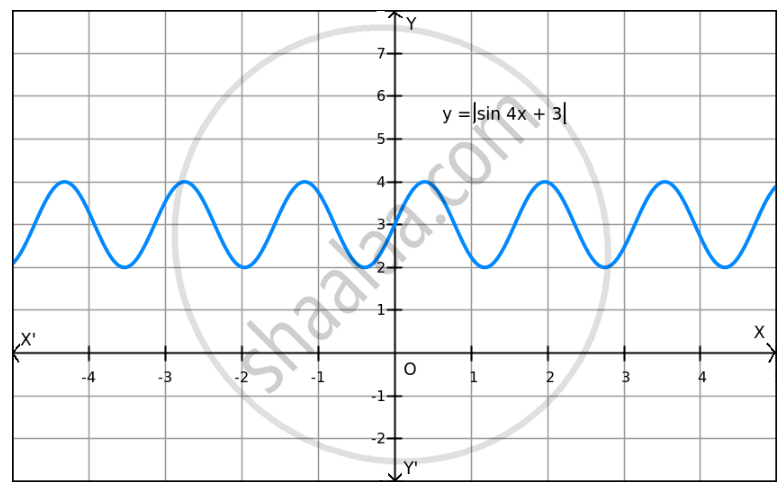Share

# F(X) = | Sin 4x+3 | on R - Mathematics

#### Question

f(x) = | sin 4x+3 | on R ?

#### Solution

Given: f(x) = $\left| \sin 4x + 3 \right|$

We know that −1 $\leq$ sin 4x $\leq$1.

⇒ 2 $\leq$ sin 4x + 3 $\leq$4

⇒ 2 $\leq$ $\left| \sin 4x + 3 \right|$ $\leq$ 4
⇒ 2 $\leq$ f(x) $\leq$ 4Hence, the maximum and minimum values of f are 4 and 2, respectively.

Is there an error in this question or solution?